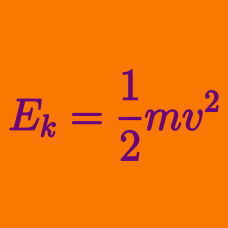Classical Mechanics

# Understanding Kinetic Energy

Two boats are having a race on a frictionless horizontal lake between two buoys separated by the distance $d.$ Boat A has a mass of $m$, while boat B has a mass of $2m$. The boats have identical sails, so the wind exerts the same constant force $F$ on each of them.

Which boat has the greater kinetic energy as it crosses the finish line?

A car traveling at $50\text{ km/h}$ will move $44$ extra meters from the moment its brakes are applied fully. If the same car travels at $100\text{ km/h}$ on the same road, how far will it move from the instant its brakes are fully applied?

An object with mass $12\text{ kg}$ is at rest on a frictionless horizontal floor. If a horizontal force $F=40\text{ N}$ is applied on the object until it has moved $10\text{ m},$ what is the object's resulting kinetic energy?

If an object initially at rest starts to fall freely, what happens to its kinetic energy?

Assume that air resistance is negligible.

Which of the following statements is/are true concerning kinetic energy?

I. Kinetic energy is a scalar quantity.

II. The kinetic energy of an object that accelerates uniformly increases linearly.

III. If a moving object changes only its direction and not speed, its kinetic energy does not change.

×